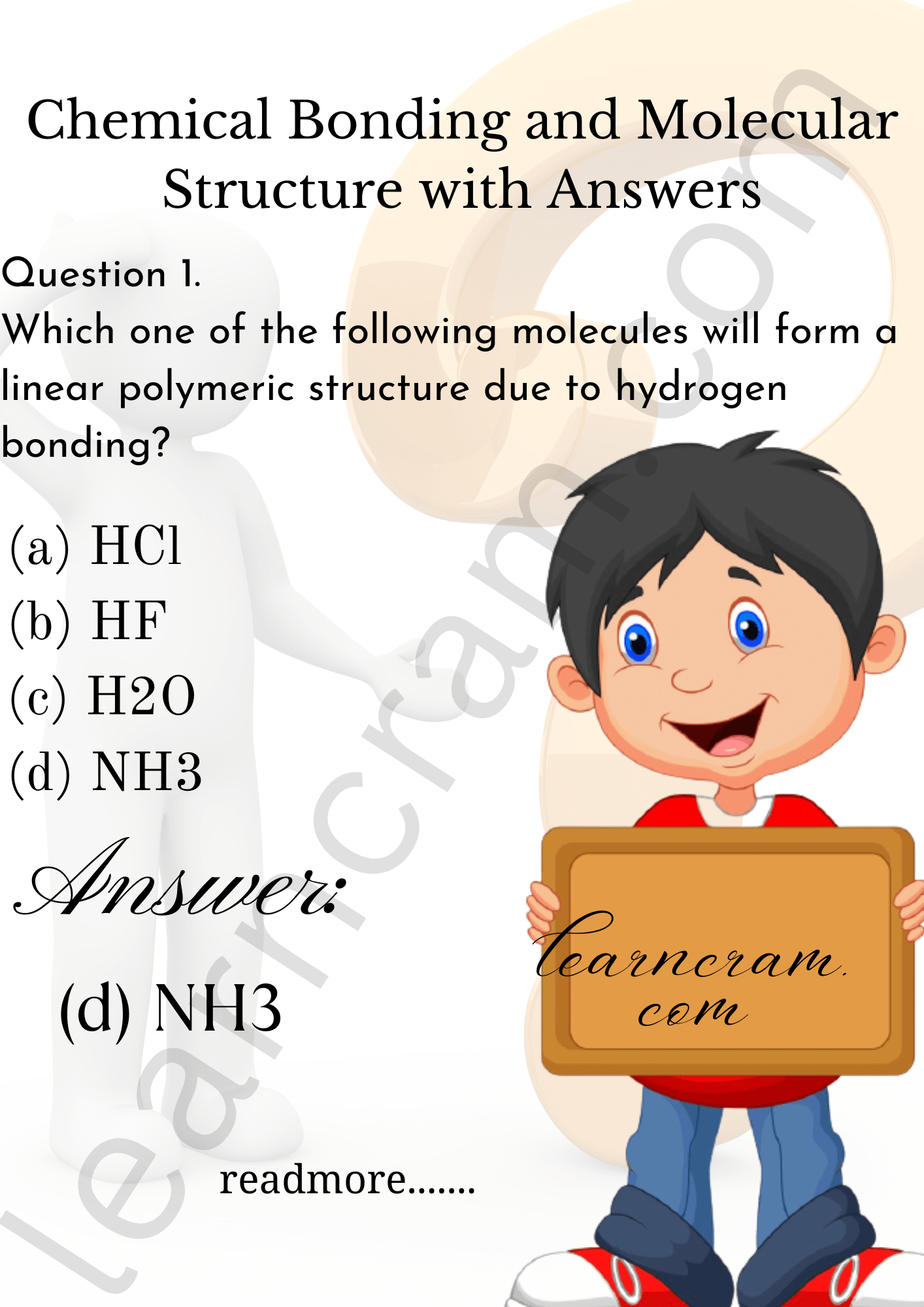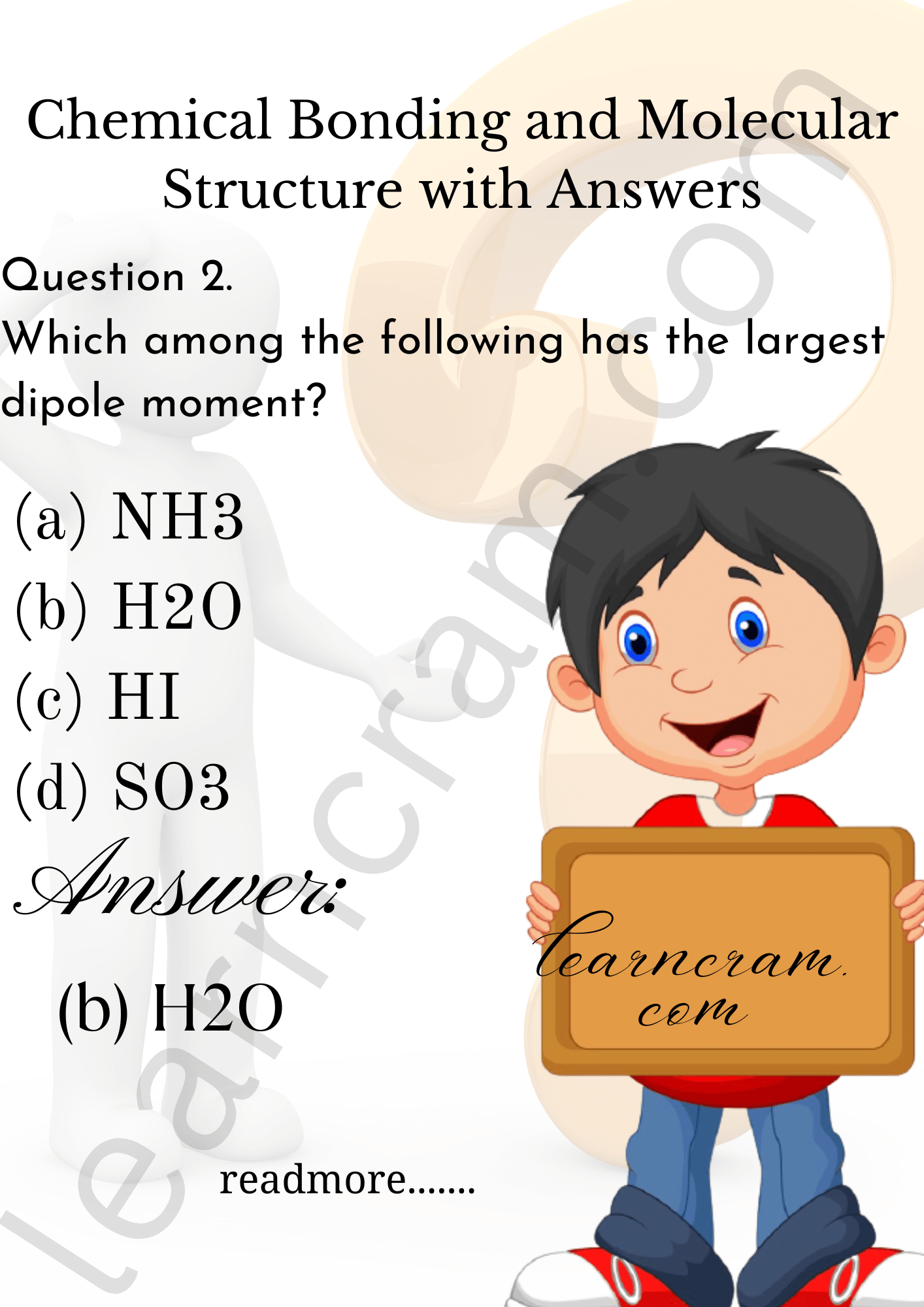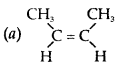# MCQ Questions for Class 11 Chemistry Chapter 4 Chemical Bonding and Molecular Structure with Answers

We have compiled the NCERT MCQ Questions for Class 11 Chemistry Chapter 4 Chemical Bonding and Molecular Structure with Answers Pdf free download covering the entire syllabus. Practice MCQ Questions for Class 11 Chemistry with Answers on a daily basis and score well in exams. Refer to the Chemical Bonding and Molecular Structure Class 11 MCQs Questions with Answers here along with a detailed explanation.

## Chemical Bonding and Molecular Structure Class 11 MCQs Questions with Answers

Multiple Choice Type Questions

Question 1.
Which one of the following molecules will form a linear polymeric structure due to hydrogen bonding?
(a) HCl
(b) HF
(c) H2O
(d) NH3Question 2.
Which among the following has the largest dipole moment?
(a) NH3
(b) H2O
(c) HI
(d) SO3Question 3.
Maximum bond angle is present in case of
(a) BBr3
(b) BCl3
(c) BF3
(d) Same in all

Question 4.
Which of the following pairs of molecules will have permanent dipole moment for both members?
(a) NO2 and CO2
(b) NO2 and O3
(c) SiF4 and CO2
(d) SiF4 and NO2

Question 5.
Which one of the following does not contain coordinate bond?
(a) BH$$_{4}^{-}$$
(b) NH$$_{4}^{-}$$
(c) CO$$_{3}^{2-}$$
(d) H3O+

Answer: (c) CO$$_{3}^{2-}$$

Question 6.
Which of the following are iso-structural?
(a) XeF2, IF~2
(b) NH3, BF3
(c) CO$$_{3}^{2-}$$, SO$$_{3}^{2-}$$
(d) PCl5, ICl5

Question 7.
Which of the following are isoelectronic and iso-structural NO$$_{3}^{-}$$, CO$$_{3}^{2-}$$, CIO$$_{3}^{-}$$, SO3
(a) NO$$_{3}^{-}$$,CO$$_{3}^{2-}$$
(b) SO3, NO$$_{3}^{-}$$
(c) CIO$$_{3}^{-}$$, CO$$_{3}^{2-}$$

Answer: (a) NO$$_{3}^{-}$$,CO$$_{3}^{2-}$$

Question 8.
Which of the following hydrocarbons has the lowest dipole moment?(b) CH3C ≡ C CH3
(c) CH3CH2C ≡ CH
(d) CH2 = CH – C ≡ CH

Answer: (b) CH3C ≡ C CH3

Question 9.
The correct order of hybridisation of the central atom in the following species: NH3, [PtCl4]2-, PCl5 and BCl3 is
(a) dsp², dsp³, sp² and sp³
(b) sp³, dsp², dsp³, sp²
(c) dsp², sp², sp³, dsp³
(d) dsp², sp³, sp², dsp³.

Answer: (b) sp³, dsp², dsp³, sp²

Question 10.
Amongst H2O, H2S, H2Se and H2Te the one with the highest boiling point is
(a) H2O because of hydrogen bonding
(b) H2Te because of higher molecular weight
(c) H2S because of hydrogen bonding
(d) H2Se because of lower molecular weight.

Answer: (a) H2O because of hydrogen bonding

Question 11.
Among KO2, AlO$$_{2}^{-}$$, BaO2 and NO$$_{2}^{+}$$ unpaired electron is present in
(a) NO$$_{2}^{+}$$ and BaO2
(b) KO2 and AIO$$_{2}^{-}$$
(c) KG2 only
(d) BaO2 only

Question 12.
Number of π bonds in Naphthalene is
(a) 6
(b) 3
(c) 4
(d) 5

Question 13.
Hydrogen bonding is not present in
(a) Glycerine
(b) Water
(c) Hydrogen sulphide
(d) Hydrogen fluoride

Question 14.
In a double bond connecting two atoms there is a sharing of
(a) 2 electrons
(b) 4 electrons
(d) 1 electron
(d) all electrons

Question 15.
Atomic orbitals of carbon in Carbon dioxide are
(a) sp hybridised
(b) sp³d hybridised
(c) sp² hybridised
(d) sp³ hybridised

Question 16.
In which of the following bond angle is maximum?
(a) NH3
(b) NH$$_{4}^{+}$$
(c) PCl5
(d) SCl2

Answer: (b) NH$$_{4}^{+}$$

Question 17.
Sulphuric acid provides a simple example of
(a) Coordinate bonds
(b) non-covalent compound
(c) non-covalent ion
(d) none of these

Question 18.
The shape and hybridisation in BF3 is
(a) sp², linear
(b) sp³d, plannar
(c) sp², planar
(d) sp³ planar

Question 19.
Bond dissociation energies of HF, HCl, HBr follow the order
(a) HCl > HBr > HF
(b) HF > HBr > HCl
(c) HF > HCl > HBr
(d) HBr > HCl > HF

Answer: (c) HF > HCl > HBr

Question 20.
Which of the following has zero dipole moment?
(a) CIF
(b) PCl3
(c) SiF4
(d) Cl3CF

Question 21.
Among the following the molecule with, highest dipole moment is
(a) CH3Cl
(b) CH2Cl2
(c) CHCl3
(d) CCl4

Question 22.
In which of the following species is the underlined carbon having sp3 hybridisation?
(a) CH3COOH
(b) CH3CH2OH
(c) CH3-CO-CH3
(d) CH2=CH-CH3

Question 23.
Which one of the following arrangements of molecules is correct on the basis of their dipole moments?
(a) BF3 > NF3 > NH3
(b) NF3 > BF3 > NH3
(c) NH3 > BF3 > NF3
(d) NH3 > NF3 > BF3
(e) NH3 = NF3 > BF3

Answer: (d) NH3 > NF3 > BF3

Question 24.
In OF2, number of bond pairs and lone pairs of electrons are respectively
(a) 2, 0
(b) 2, 8
(c) 2, 10
(d) 2, 9

Question 25.
In NO$$_{3}^{-}$$ ion, the number of bond pairs and lone pairs of electrons on N atoms are
(a) 2, 2
(b) 3, 1
(c) 1, 3
(d) 4, 0

Question 26.
In which of the following pairs the two species have identical bond order?
(a) N$$_{2}^{-}$$, O$$_{2}^{2-}$$
(b) N$$_{2}^{+}$$, O$$_{2}^{-}$$
(c) N$$_{2}^{-}$$, O$$_{2}^{+}$$
(d) O$$_{2}^{+}$$, N$$_{2}^{2-}$$

Answer: (c) N$$_{2}^{-}$$, O$$_{2}^{+}$$

Question 27.
The bond order of O$$_{2}^{-}$$ is
(a) 0.5
(b) 1.5
(c) 2.5
(d) 3.5.

Question 28.
Which of the following is not paramagnetic?
(a) N$$_{2}^{+}$$
(b) CO
(c) O$$_{2}^{-}$$
(d) NO.

Question 29.
The number of antibonding electron pairs in O$$_{2}^{2-}$$ molecular ion on the basis of M.O. theory (At. No. of O = 8) is
(a) 2
(b) 3
(c) 4
(d) 5

Question 30.
Main axis of a diatomic molecule is Z. Atomic orbitals px and py overlap to form which of the following orbital?
(a) π-molecular orbital
(b) σ-molecular orbital
(c) δ-molecular orbital
(d) no bond will form

Answer: (d) no bond will form

Fill in the Blanks

Question 1.
H2O shows ……………….. hybridization, whereas BeF2 shows hybridization.

Question 2.
02 has ………………. unpaired electron, whereas O2 has unpaired electrons.

Question 3.
Out of C2H6, C2H4, CH4, C2H2 it is only ……………….. which shows sp hybridization.

Question 4.
CaC2 molecule has ……………….. bonds in it.

Question 5.
Atomic orbitals of carbon in carbon dioxide are ……………….. hybridized.

Question 6.molecule contains ……………….. σ bonds and ……………….. π bonds.

Question 7.
XeF2 and CO2 molecules have ……………….. shapes.

Question 8.
PF5 has ……………….. geometry whereas IF5 has geometry.

Question 9.
The lone pair-lone pair repulsion are ……………….. than lone pair-bond pair repulsions.

Question 10.
A bond formed between two atoms by the overlap of their atomic orbitals along the internuclear axis is called a ……………….. bond.

True/False Statements

Question 1.
Bond order of O2 is greater than that of O$$_{2}^{+}$$

Question 2.
Bond length in N2 is greater than that of O2.

Question 3.
O2 is paramagnetic whereas O$$_{2}^{2-}$$ is diamagnetic.

Question 4.
BF3 is triangular planar, whereas NH3 is pyramidal.

Question 5.
HF shows hydrogen bonding but HBr does not.

Question 6.
Both benzene and carbon tetrachloride are non-polar.

Question 7.
The presence of polar bonds in a polyatomic molecule suggests that the molecule has non-zero dipole moment.

Question 8.
Whereas NH3 has one lone pair of electrons, NH$$_{4}^{+}$$ ion does not have any.

Question 9.
The dipole moment of NF3 is more than NH3.

Question 10.
Out of sp³, sp², sp hybrid orbitals, sp³ has maximum s-character.

Match Type Questions

 Column I Column II (a) Stable octet rule (i) unpaired electrons (b) NaCl (ii) sp³ hybridization (c) CH4 (iii) Ionic compound (d) Paramagnetism (iv) Lewis

 Column I Column II (a) Stable octet rule (iv) Lewis (b) NaCl (iii) Ionic compound (c) CH4 (ii) sp³ hybridization (d) Paramagnetism (i) unpaired electrons

 Column I Column II (a) SnCl2 (i) Linear (b) CO$$_{3}^{2-}$$ (ii) V-shape (c) HgCl2 (iii) Trigonal pyramidal (d) H3O+ (iv) Triangular planar

 Column I Column II (a) SnCl2 (ii) V-shape (b) CO$$_{3}^{2-}$$ (iii) Trigonal pyramidal (c) HgCl2 (i) Linear (d) H3O+ (iv) Triangular planar

 Column I Column II (a) O-nitrophenol (i) duplet (b) H2O (ii) Intramolecular H-bond (c) Benzene (iii) Intermolecular H-bond (d) H2 (iv) Triangular planar

 Column I Column II (a) O-nitrophenol (ii) Intramolecular H-bond (b) H2O (iii) Intermolecular H-bond (c) Benzene (iv) Non-polar (d) H2 (i) duplet

 Column I Column II (a) ICl$$_{2}^{2}$$ (i) V-shape (b) NH$$_{2}^{-}$$ (ii) Linear (c) NH$$_{4}^{+}$$ (iii) Tetrahedral (d) [PtCl4]2- (iv) square planar
 Column I Column II (a) ICl$$_{2}^{2}$$ (ii) Linear (b) NH$$_{2}^{-}$$ (i) V-shape (c) NH$$_{4}^{+}$$ (iii) Tetrahedral (d) [PtCl4]2- (iv) square planar